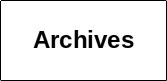Personal tools

######You are here: Home / Optimal Definition of Class Intervals for Frequency Tables

WILLIAM FULL, ROBERT EHRLICH and STEPHEN KENNEDY (1983)

# Optimal Definition of Class Intervals for Frequency Tables

Particulate Science and Technology, 1(3):281-293.

Data sets are often analyzed in the form of collections of frequency tables (or percentiles derived from equivalent cumulative frequency distributions). Decisions concerning the number of intervals and interval width obviously affect the quality of the data in subsequent analysis. Relying on the basic concepts of information theory, a procedure is presented which evaluates the relative information content of a set of frequency data when subdivided in various manners. Maximum information is always preserved when “maximum entropy” histograms (with unequal class intervals) are used. Evaluation of several schemes of frequency table subdivision (phi-based arithmetic, log arithmetic, Z-score, log Z-score, maximum entropy) indicates that, surprisingly, collections of equal interval phi-based frequency tables contain the least information. Additionally, the concept of the relative entropy of a given collection of frequency tables is defined. The relative entropy is useful as a feature extractor wherein several collections of data with potentially similar information can be compared. An example of using the relative entropy as a feature extractor is given in shape analysis where the choice of which harmonic(s) represents the greatest shape differences need to be defined.

Document Actions

List
• ###### Publication title and author(s)
Find PDF

RBINS private PDFs
e-ressources